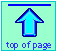Note: this document will print in an appropriately modified format (12 pages)

1  What is a spontaneous process?

Processes that proceed in a definite direction when left to themselves and in the absence of any attempt to drive them in reverse — are known as natural processes or spontaneous changes. In many cases our everyday life experiences teach us the direction in which spontaneous change can occur, and anything that runs counter to these expectations is immediately sensed as weird. In other cases, including that of most chemical change, we often have no obvious guidelines, and must learn how to apply the laws of thermodynamics which ultimately govern all spontaneous change.

A non-natural process: Steinberg's famous New Yorker cartoonSaul Steinberg (1914-1999) was one of America's most admired artists whose unique and imaginative cartoon style graced the pages of the New Yorker magazine for more than 60 years.

In order to answer the above question, let's begin by thinking about the outcomes of the following four ooooexperiments, each of which illustrates a natural process that proceeds spontaneously only in a single direction.

Experiment 1. A stack of one hundred coins is thrown into the air. After they have come to rest on the floor, the numbers that land “heads up” and “tails up” are noted.

Net change: ordered coins → randomized coins
(roughly equal numbers of heads and tails.)

Energetics: no relevant net change in energy

Why it does not go in reverse: Simple statistics shows that the probability that the coins will land in one particular arrangement of the huge number that are possible is vanishingly small.

Experiment 2. Two identical blocks of copper, one at 200°C and the other at 100°C, are brought into contact in a thermally-insulated environment. Eventually the temperatures of both blocks reach 150°C.

Net change: block1 (200°) + block2 (100°) → combined blocks (150°)

Energetics: Heat (randomized molecular kinetic energy) flows from the warmer block to the cooler one until their temperatures are identical.

Why it does not go in reverse: Dispersal of kinetic energy amongst the copper atoms is a random process; the chances that such a process would lead to a non-uniform sharing of the energy are even smaller than in the case of the 100 coins because of the much greater number (around 1022) of particles involved.

Experiment 3. A book or some other solid object is held above a table top, and is then allowed to fall.

Net change: book in air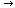book on table top; potential energyorganized kinetic energythermal energy.

Energetics: At the instant just before the end of its fall, the potential energy the object acquired when it was raised will exist entirely as kinetic energy mv2/2 in which m is the mass of the object and v is its velocity. Each atom of which the object is composed will of course possess a proportionate fraction of this energy, again with its principal velocity component pointing down. Superimposed on this, however, will be minute thermal displacements that vary randomly in magnitude and direction from one instant to the next. The sum total of these constitutes the thermal energy contained in the object.

When the object strikes the table top, its motion ceases and we say its kinetic energy is zero. Energy is supposed to be conserved, so where did it disappear to? The shock of impact has resulted in its dispersal into greatly augmented thermal motions of the atoms, both of the object itself and of the area of the table top where the impact occurred. In other words, the kinetic energy of organized motion the object had just before its motion stopped has been transformed into kinetic energy of random or disorganized motion (thermal energy) which spreads rapidly away from the point of impact.

Why it does not go in reverse: Once the kinetic energy of the book has been dispersed amongst the molecules of the book and the table top, the probabability of these randomized motions reappearing at the surface where the two objects are in contact and then acting in concert to propel the object back into the air) is negligible.

Experiment 4. One mole of gas, initially at 300 K and 2 atm pressure, is allowed to expand to double its volume, keeping the temperature constant.

Net change: Increase in volume of gas.

Energetics: No change in energy if the gas behaves ideally.

Why it does not go in reverse: Simple statistics: the probabiliy that N randomly moving objects (flies in a bottle, for example,) will at any time all be located in one half of the container is (1/2)N. For chemically-significant values of N (1020, say) this probability is indistinguishable from zero.

All of the changes described above take place spontaneously, meaning that

• Once they are allowed to commence, they will proceed to the finish without any outside intervention.
• It would be inconceivable that any of these changes could occur in the reverse direction (that is, be undone) without changing the conditions or actively disturbing the system in some way.

What determines the direction in which spontaneous change will occur? It is clearly not a fall in the energy, since in most cases cited above the energy of the system did not change. Even in the case of the falling book, in which the potential energy of the system (the book) falls, energy is conserved overall; if there is no net loss of energy when these processes operate in the forward or natural direction, it would not require any expenditure of energy for them to operate in reverse. In other words, contrary to what all too many people appear to believe,

The direction of a spontaneous process is not governed by the energy change...

... and thus the First Law of Thermodynamics cannot predict the direction of a natural process.

2  Direction through disorder

In our examination of the processes described above, we saw that although the total energy of the system and the surroundings (and thus, of the world) is unchanged, there is something about the world that has changed, and this is its degree of disorder.

After coins have been tossed or cards shuffled, the final state is invariably one of greater disorder. Similarly, the molecules of a gas can occupy a larger number of possible positions in space if the volume is larger, so the expansion of a gas is similarly accompanied by an increase in randomness.

### A closer look at disorder: microstates and macrostates

How can we express disorder quantitatively? From the example of the coins, you can probably see that simple statistics plays a role: the probability of obtaining three heads and seven tails after tossing ten coins is just the ratio of the number of ways that ten different coins can be arranged in this way, to the number of all possible arrangements of ten coins.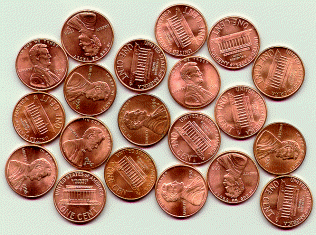Using the language of molecular statistics, we say that a collection of coins in which a given fraction of its members are heads-up constitutes a macroscopic state of the system. Since we don’t care which coins are heads-up, there are clearly numerous configurations of the individual coins which can result in this “macrostate”. Each of these configurations specifies a microscopic state of the system.

The greater the number of microstates that correspond to a given macrostate,
the greater the probability of that macrostate.

To see what this means, consider the possible outcomes of a toss of four coins:

 macrostate ways probability microstates 0 heads 1 1/16 TTTT 1 head 4 4/16 = 1/4 HTTT THTT TTHT TTTH 2 heads 6 6/16 = 3/8 HHTT HTHT HTTH THHT TTHH THTH 3 heads 4 4/16 = 1/4 HHHT HTHH HHTH THHH 4 heads 1 1/16 HHHH

A toss of four coins will yield one of the five outcomes (macrostates) listed in the leftmost column of the table. The second column gives the number of “ways”— that is, the number of head/tail configurations of the set of coins (the number of microstates)— that can result in the macrostate. The probability of a toss resulting in a particular macrostate is proportional to the number of microstates corresponding to the macrostate, and is equal to this number, divided by the total number of possible microstates (in this example, 24 =16). An important assumption here is that all microstates are equally probable; that is, the toss is a “fair” one in which the many factors that determine the trajectory of each coin operate in an entirely random way.

Disorder is more probable than order because there are so many more ways of achieving it. Thus coins and cards tend to assume random configurations when tossed or shuffled, and socks and books tend to become more scattered about a teenager’s room during the course of daily living. But there are some important differences between these large-scale mechanical, or macro systems, and the collections of sub-microscopic particles that constitute the stuff of chemistry, and which we will refer to here generically as molecules. Molecules, unlike macro objects, are capable of accepting, storing, and giving up energy in tiny amounts (quanta), and act as highly efficient carriers and spreaders of thermal energy as they move around. Thus, in chemical systems,

1. We are dealing with huge numbers of particles. This is important because statistical predictions are always more accurate for larger samples. Thus although for the four tosses there is a good chance (62%) that the H/T ratio will fall outside the range of 0.45 - 0.55, this probability becomes almost zero for 1000 tosses. To express this in a different way, the chances that 1000 gas molecules moving about randomly in a container would at any instant be distributed in a sufficiently non-uniform manner to produce a detectable pressure difference between the two halves of a container will be extremely small. If we increase the number of molecules to a chemically significant number (around 1020, say), then the same probability becomes indistinguishable from zero.
2. Once the change begins, it proceeds spontaneously. That is, no external agent (a tosser, shuffler, or teen-ager) is needed to keep the process going. Gases will spontaneously expand if they are allowed to, and reactions, one started, will proceed toward equilibrium.
3. Thermal energy is continually being exchanged between the particles of the system, and between the system and the surroundings. Collisions between molecules result in exchanges of momentum (and thus of kinetic energy) amongst the particles of the system, and (through collisions with the walls of a container, for example) with the surroundings.
4. Thermal energy spreads rapidly and randomly throughout the various energetically accessible microstates of the system. The direction of spontaneous change is that which results in the maximum possible spreading and sharing of thermal energy.

The importance of these last two points is far greater than you might at first think, but to fully appreciate this, you must recall the various ways in which thermal energy is stored in molecules— hence the following brief review.

### From coins to molecules: the spreading of energy

Thermal energy is the portion of a molecule's energy that is proportional to its temperature, and thus relates to motion at the molecular scale. What kinds of molecular motions are possible? For monatomic molecules, there is only one: actual movement from one location to another, which we call translation. Since there are three directions in space, all molecules possess three modes of translational motion.

For polyatomic molecules, two additional kinds of motions are possible. One of these is rotation; a linear molecule such as CO2 in which the atoms are all laid out along the x-axis can rotate along the y- and z-axes, while molecules having less symmetry can rotate about all three axes. Thus linear molecules possess two modes of rotational motion, while non-linear ones have three rotational modes.

Finally, molecules consisting of two or more atoms can undergo internal vibrations. For freely moving molecules in a gas, the number of vibrational modes or patterns depends on both the number of atoms and the shape of the molecule, and it increases rapidly as the molecule becomes more complicated.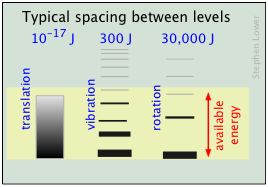The relative populations of the translational, rotational and vibrational energy states of a typical diatomic molecule are depicted by the thickness of the lines in this schematic (not-to-scale!) diagram. The colored shading indicates the total thermal energy available at a given temperature. The numbers at the top show order-of-magnitude spacings between adjacent levels. It is readily apparent that virtually all the thermal energy resides in translational states.

Notice the greatly different spacing of the three kinds of energy levels. This is extremely important because it determines the number of energy quanta that a molecule can accept, and, as the following illustration shows, the number of different ways this energy can be distributed amongst the molecules.

The spacing of molecular energy states becomes closer as the mass and number of bonds in the molecule increases, so we can generally say that the more complex the molecule, the greater the density of its energy states.

This is a feature of the particle-in-a-box model, which predicts that the separation of the energy states of a gas confined within a box depends on the inverse square of the box length (and on the inverse of the particle mass as well.)

3  Quantum states, microstates, and energy spreading

At the atomic and molecular level, all energy is quantized; each particle possesses discrete states of kinetic energy and is able to accept thermal energy only in packets whose values correspond to the energies of one or more of these states. Polyatomic molecules can store energy in rotational and vibrational motions, and all molecules (even monatomic ones) will possess tranlational kinetic energy (thermal energy) at all temperatures above absolute zero. The energy difference between adjacent translational states is so minute (roughly 10–30 J) that translational kinetic energy can be regarded as continuous (non-quantized) for most practical purposes.

A very important point to bear in mind is that the number of discrete microstates that can be populated by an arbitrary quantity of energy depends on the spacing of the states. As a very simple example, suppose that we have two molecules (depicted by the orange dots) in a system whose total available thermal energy is indicated by the yellow shading: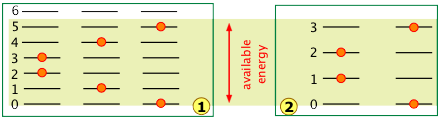In system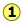, the available energy is sufficient to populate three different microstates in which the two molecules are distributed over the quantized levels 0 through 5.

Owing to the wider spacing of the quantum levels in system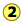, the same amount of energy is able to excite only four quantum levels, reducing the number of possible microstates to two.

It's actually a bit more complicated than this, because simple exchange of molecules between the same two levels increases the number of microstates.

Suppose that we have a system consisting of three molecules and three quanta of thermal (kinetic) energy to share among them. We can give all the kinetic energy to any one molecule, leaving the others with none, we can give two units to one molecule and one unit to another, or we can share out the energy equally and give one unit to each molecule. All told, there are ten possible ways of distributing three units of energy among three identical molecules as shown here: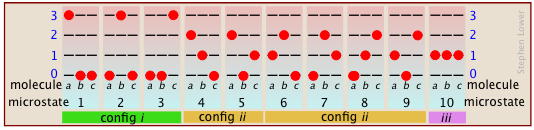Each of these ten possibilities represents a distinct microstate that will describe the system at any instant in time. Those microstates that possess identical distributions of energy among the accessible quantum levels (and differ only in which particular molecules occupy the levels) are known as configurations. Because all microstates are equally probable, the probability of any one configuration is proportional to the number of microstates that can produce it. Thus in the system shown above, the configuration labeled ii will be observed 60% of the time, while iii will occur only 10% of the time.

#### huge ... Huge ... HUGE ... HUGE !

As the number of molecules and the number of quanta increases, the number of accessible microstates grows explosively; if 1000 quanta of energy are shared by 1000 molecules, the number of available microstates will be around 10600— a number that greatly exceeds the number of atoms in the observable universe! The number of possible configurations (as defined above) also increases, but in such a way as to greatly reduce the probability of all but the most probable configurations. Thus for a sample of a gas large enough to be observable under normal conditions, only a single configuration (energy distribution amongst the quantum states) need be considered; even the second-most-probable configuration can be neglected.

The bottom line: any collection of molecules large enough in numbers to have chemical significance will have its therrmal energy distributed over an unimaginably large number of microstates. The number of microstates increases exponentially as more energy states ("configurations" as defined above) become accessible owing to

• Addition of energy quanta (higher temperature),
• Increase in the number of molecules (resulting from dissociation, for example).
• the volume of the system increases (which decreases the spacing between energy states, allowing more of them to be populated at a given temperature.)

Energy is conserved; if you lift a book off the table, and let it fall, the total amount of energy in the world remains unchanged. All you have done is transferred it from the form in which it was stored within the glucose in your body to your muscles, and then to the book (that is, you did work on the book by moving it up against the earth’s gravitational field). After the book has fallen, this same quantity of energy exists as thermal energy (heat) in the book and table top.

What has changed, however, is the availability of this energy. Once the energy has spread into the huge number of thermal microstates in the warmed objects, the probabiliy of its spontaneously (that is, by chance) becoming un-dispersed is essentially zero. Thus although the energy is still “there”, it is forever beyond utilization or recovery.

The profundity of this conclusion was recognized around 1900, when it was first described as the “heat death” of the world. This refers to the fact that every spontaneous process (essentially every change that occurs) is accompanied by the “dilution” of energy. The obvious implication is that all of the molecular-level kinetic energy will be spread out completely, and nothing more will ever change. Not a happy thought!

### Why do gases tend to expand but never contract?

Everybody knows that a gas, if left to itself, will tend to expand so as to fill the volume within which it is confined completely and uniformly. What “drives” this expansion? At the simplest level it is clear that with more space available, random motions of the individual molecules will inevitably disperse them throughout the space. But as we mentioned above, the allowed energy states that molecules can occupy are spaced more closely in a larger volume than in a smaller one. The larger the volume available to the gas, the greater the number of energy states (and thus microstates) its thermal energy can occupy. Since all such microstates within the thermally accessible range of energies are equally probable, the expansion of the gas can viewed as a consequence of the tendency of energy to be spread and shared as widely as possible. Once this has happened, the probability that this sharing of energy will reverse itself (that is, that the gas will spontaneously contract) is so minute as to be unthinkable.

The same can in fact be said for even other highly probable distributions, such as having 49.999% of the molecules in the left half of the container and 50.001% in the right half. Even though the number of possible configurations that would yield this distribution of molecules is uncountably great, it is essentially negligible compared to the number that would correspond to an exact 50-percent distribution.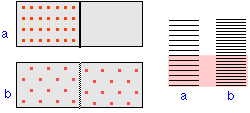The illustration represents the allowed thermal energy states of an ideal gas. The larger the volume in which the gas is confined, the more closely-spaced are these states, resulting in a huge increase in the number of microstates into which the available thermal energy can reside; this can be considered the origin of the thermodynamic "driving force" for the spontaneous expansion of a gas.

### Why heat flows from hot to cold

Just as gases spontaneously change their volumes from “smaller-to-larger”, the flow of heat from a warmer body to a cooler always operates in the direction “warmer-to-cooler” because this allows thermal energy to occupy a larger number of energy states as new ones are made available by bringing the cooler body into contact with the warmer one; in effect, the thermal energy becomes more “diluted”.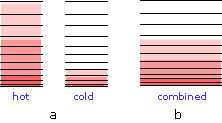When two bodies at different temperaures are placed in thermal contact, thermal energy flow from the warmer into the cooler one until they reach the same temperature.

(a) Schematic depiction of the thermal energy states in two separated identical bodies at different temperatures (indicated by shading.)

(b) When the bodies are brought into thermal contact, thermal energy flows from the higher occupied levels in the warmer object into the unoccupied levels of the cooler one until equal numbers are occupied .

As you might expect, the increase in the amount of energy spreading and sharing is proportional to amount of heat transferred q, but there is one other factor involved, and that is the temperature at which the transfer occurs. When a quantity of heat q passes into a system at temperature T, the degree of dilution of the thermal energy is given by q /T

To understand why we have to divide by the temperature, consider the effect of very large and very small values of T in the denominator. If the body receiving the heat is initially at a very low temperature, relatively few thermal energy states are occupied, so the amount of energy spreading can be very great. Conversely, if the temperature is initially large, the number of new thermal energy states that become occupied will be negligible compared to the number already active.

5   Energy spreading and sharing in chemical reactions

When a chemical reaction takes place, two kinds of changes relating to thermal energy are involved:

1. The ways that thermal energy can be stored within the reactants will generally be different from those for the products. For example, in the reaction H2 → 2 H, the reactant dihydrogen possesses vibrational and rotational energy states, while the atomic hydrogen in the product has translational states only— but the total number of translational states in two moles of H is twice as great as in one mole of H2. Because of their extremely close spacing, translational states are the only ones that really count at ordinary temperatures, so we can say that thermal energy can become twice as diluted (“spread out”) in the product than in the reactant. If this were the only factor to consider, then dissociation of dihydrogen would always be spontaneous and H2 would not exist.
2. In order for this dissociation to occur, however, a quantity of thermal energy (heat) q = ΔU must be taken up from the surroundings in order to break the H–H bond. In other words, the ground state (the energy at which the manifold of energy states begins) is higher in H, as indicated by the vertical displacement of the right half in each of the four panels below.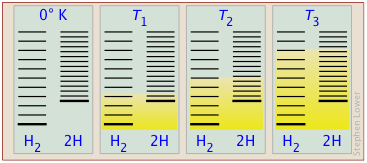Energy levels in H2 and 2 H. The number of thermally accessible energy states (indicated by the shading in the diagram below) increases with temperature, but because 2 moles of H possess twice as many translational states as one mole of H2, dissociation becomes increasingly favored at higher temperatures as more of these H states become thermally accessible.

This argument can be generalized to all molecules, illustrating an important principle:

At high temperatures, all molecules spontaneousy absorb heat and dissociate into gaseous atoms

The ability of energy to spread into the product molecules is constrained by the availability of sufficient thermal energy to produce these molecules. This is where the temperature comes in. At absolute zero the situation is very simple; no thermal enegy is available to bring about dissociation, so the only component present will be dihydrogen.

• As the temperature increases, the number of populated energy states rises, as indicated by the shading in the diagram. At temperature T1, the number of populated states of H2 is greater than that of 2H, so some of the latter will be present in the equilibrium mixture, but only as the minority component.
• At some temperature T2 the numbers of populated states in the two components of the reaction system will be identical, so the equilibrium mixture will contain H2 and “2H” in equal amounts; that is, the mole ratio of H2/H will be 1:2.
• As the temperature rises to T3 and above, we see that the number of energy states that are thermally accessible in the product begins to exceed that for the reactant.

The result is exactly what the LeChâtelier Principle predicts: the equilibrium state for an endothermic reaction is shifted to the right at higher temperatures.

This is all very well for helping you understand the direct connection between energy spreading when a chemical reaction occurs, but it is of little help in achieving our goal of predicting the direction and extent of chemical change. For this, we need to incorporate the concept of energy spreading into thermodynamics.